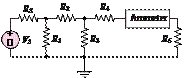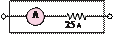### Create an Account

Already have account?

### Forgot Your Password ?

Home / Questions / An ammeter is used as shown in Figure P278 The ammeter model consists of an ideal ammeter ...

# An ammeter is used as shown in Figure P278 The ammeter model consists of an ideal ammeter in series with a resistance The ammeter model is placed in the branch as shown in the figure Find the

An ammeter is used as shown in Figure P2.78. The ammeter model consists of an ideal ammeter in series with a resistance. The ammeter model is placed in the branch as shown in the figure. Find the current through R5  both with and without the ammeter in the circuit for

the following values, assuming that R= 20 Q,

R1 = 800 Q, R2 = 600 QR3 = 1.2 kQR4 = 150 Q, and VS  = 24 V.

a.  R5 = 1 kQ

b. R5 = 100 Q

c.    R5 = 10 Q

d.    R5 = 1 Q

Ammeter internal modelFigure P2.78

Jun 19 2020 View more View Less

#### Answer (Solved)Subscribe To Get Solution